Electron. J. Differential Equations, Vol. 2020 (2020), No. 98, pp. 1-29.

### Nehari manifold approach for fractional p(.)-Laplacian system involving concave-convex nonlinearities Reshmi Biswas, Sweta Tiwari

Abstract:
In this article, using Nehari manifold method we study the multiplicity of solutions of the nonlocal elliptic system involving variable exponents and concave-convex nonlinearities,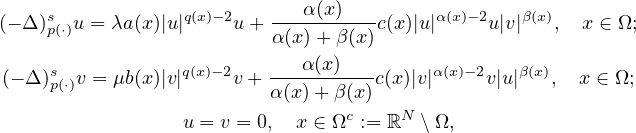where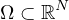,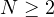is a smooth bounded domain,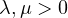are parameters, and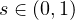. We show that there exists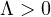such that for all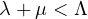, this system admits at least two non-trivial and non-negative solutions under some assumptions on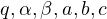.

Submitted November 2, 2019. Published September 23, 2020.
Math Subject Classifications: 35J48, 35J50, 35R11.
Key Words: Nonlocal problem with variable exponents; elliptic system; Nehari manifold; fibering map; concave-convex nonlinearities.

Show me the PDF file (445 KB), TEX file for this article.Reshmi Biswas Department of Mathematics IIT Guwahati, Assam 781039, India email: b.reshmi@iitg.ac.in Sweta Tiwari Department of Mathematics IIT Guwahati, Assam 781039, India email: swetatiwari@iitg.ac.in